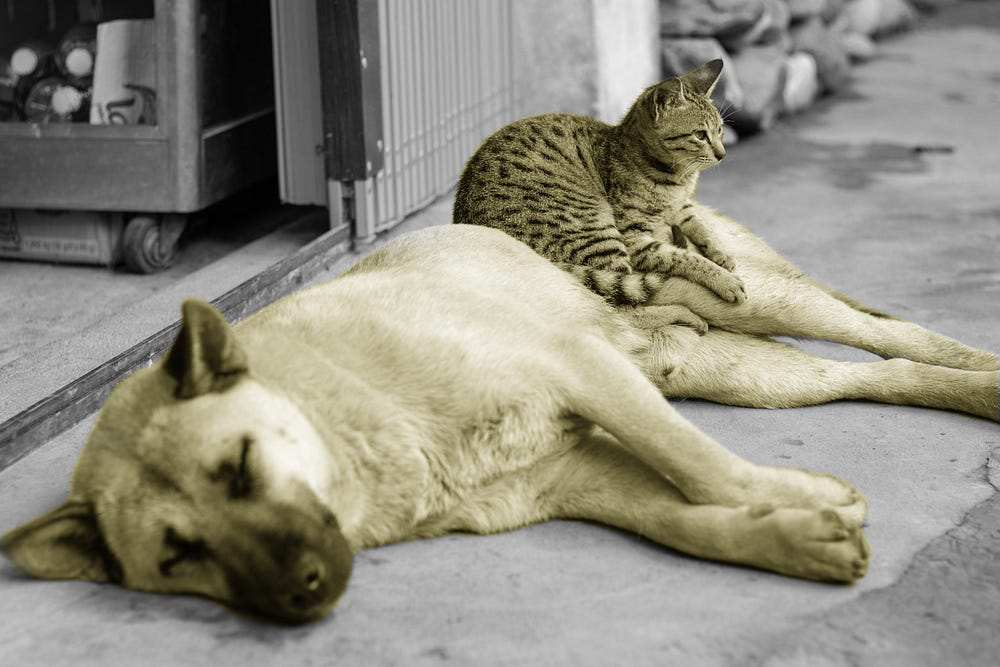# Build a deep learning model to classify images using Keras and TensorFlow 2.0

Original article can be found here (source): Deep Learning on Medium# Adding Layers to the Neural Network

We’ll use the vector we obtained above as the input for the neural network by using the `Dense` function in Keras.

The first parameter it takes is `units`, which is the number of nodes in the hidden layer. The optimum number of `units` can be determined via experimentation. The second parameter is the activation function. The `ReLu` activation function is usually used in this layer.

The flattened feature map is passed to the CNN. The input layer, the fully connected layer, and the output layer are involved in this process. The fully connected layer is the same as the hidden layer in artificial neural networks, only that now it’s fully connected. The predicted image classes are obtained from the output layer.

The network computes the predictions as well as the errors in the prediction process. The network improves its predictions via backpropagation of the errors. The final result is a number between zero and one. This number represents the probability of each class.

`classifier.add(Dense(units = 128, activation='relu'))`

We’re now ready to add the output layer. In this layer, we’ll use the sigmoid activation function since we expect a binary outcome. If we expected more than two possible outcomes, we’d have used the softmax function.
The `units` here is 1 since we expect just the predicted probabilities of the classes.

`classifier.add(Dense(units=1, activation='sigmoid'))`

We now have all the layers for the deep learning model in place. However, before we can start training the model, we have to ensure that we’re reducing the errors that occur during the training process. This maximizes the chances of getting good results from the model. Therefore, in the next step, we’ll implement a strategy that will reduce errors during training.

# Compiling the CNN

Compiling the CNN is done using the `compile` function. The function expects three arguments:

• the optimizer,
• the loss function
• the performance metrics

We’ll apply gradient descent as an optimizer for the model. In this case, the `binary_crossentropy` loss function is most appropriate since this is a binary classification problem.

Gradient descent is an optimization strategy that works to reduce errors during the training process in order to get to the point where the error is least. This is achieved by finding the point where the cost function is at its minimum. This is known as the local minimum and is found by differentiating the slope at a specific point and descending into the minimum of the cost function. Here, we’ll use the popular Adam optimizer.

`classifier.compile(optimizer='adam', loss='binary_crossentropy',metrics=['accuracy'])`

We’re now sure that errors will be handled properly during training, we’re ready to fit the classifier to the training images.

# Fitting the CNN

Before we can fit the CNN, we’ll pre-process the images using Keras in order to reduce overfitting. This process is known as `image augmentation`. We’ll use the `ImageDataGenerator` function for this purpose.

`from keras.preprocessing.image import ImageDataGenerator`

The function will rescale, zoom, shear, and flip the images. The `rescale` parameter rescales the images pixel values between zero and one. `horizontal_flip=True` flips the images horizontally.

`train_datagen = ImageDataGenerator(rescale=1./255, shear_range=0.2, zoom_range=0.2, horizontal_flip=True)`

After that, we’ll also need to rescale the test data images using `ImageDataGenerator`.

`test_datagen = ImageDataGenerator(rescale=1./255)`

Next, we’ll create a training set using `train_datagen`. Use `flow_from_directory` to obtain the images from your current working directory. Pass in the path as the first parameter and `target_size` as the second parameter.

The `target_size` is the size of the image. We’ll use 256×256 since we’ve already specified it above. `batch_size` is the number of images that must have passed through the network so that the weights are updated. We specify `class_mode` as binary since this is indeed a binary classification problem.

Run the following command to load in the training images. Since our Notebook and the `training_set` are in the same folder, the images will be loaded without any errors.

`training_set = train_datagen.flow_from_directory('training_set', target_size=(256, 256), batch_size=32, class_mode='binary')`

Now we’ll create a test set with similar parameters as above. Again, since our Jupyter Notebook and the `test_set` are in the same folder, the test set images will be loaded without any errors.

`test_set = test_datagen.flow_from_directory('test_set', target_size=(256, 256), batch_size=32, class_mode='binary')`

Fitting the classifier to the training set is done by calling the
`fit_generator` function on the `classifier` object. We pass in the training set as the first argument. `steps_per_epoch` is the number of steps obtained from the generator before one epoch is finished. `epochs` is the number of iterations used to train the CNN on. `validation_steps` is the total number of steps to validate before stopping.

`classifier.fit_generator(training_set, steps_per_epoch=40, epochs=25, validation_data=test_set, validation_steps=1000)OutputEpoch 1/2540/40 [==============================] - 7341s 1s/step - loss: 8.0578 - acc: 0.5000 - val_loss: 8.0575 - val_acc: 0.5001<keras.callbacks.History at 0x7f87a8efb7f0>`

To recap, in this step, we loaded in the training and test images, pre-processed them, and fitted the training set to the model we created. It’s now time to test the model on an unseen test image.